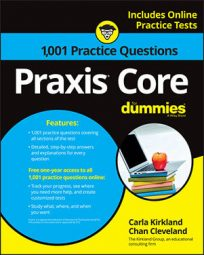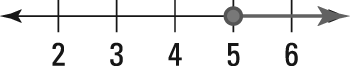##### Praxis Core: 1,001 Practice Questions For DummiesProblems involving inequalities on the Praxis Core exam will usually require you to do some math—but they may also involve recognizing the symbols on a number line.

In the following practice questions, you start by solving an inequality, and then you need to match the inequality to its appropriate graph on a number line.

## Practice questions

1. If9j − 13 ≥ 4j + 17

which of the following CANNOT be the value of j?

A. 6 B. 7 C. 5 D. 9 E. 8

2. Which of the following graphs represents the inequality x > 4?A.B.

C.

D.

E.

1. The correct answer is Choice (C). First, get the j terms on one side of the inequality by subtracting either 9j or 4j from both sides. Then undo everything that's being done to j by performing opposite operations on both sides:

The last inequality has j on one side and by itself on that side, so it's the solution. Because the value of j is either 6 or something greater than 6, only Choice (C), 5, cannot be the value of j.

2. The correct answer is Choice (E). Choice (E) is a graph in which the point representing 4 has a clear circle around it, indicating that 4 is a boundary for a region of the graph but isn't part of the region. The line is darkened infinitely to the right of 4, representing all numbers that are greater than 4.

A darkened circle indicates that a number is included in a set, and it's used when "or equal to"

is involved in an inequality. Choice (D) is the graph for

because the darkened circle shows that 4 is included in the solution set.